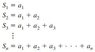# Sequences and Series

Bashyboy
Hello,

I am curious to know that if we take some seqence, $a_n$, and take the limit as the the terms of the sequence goes to infinity, will the sequence head towards the same value that the the sum of the infinite amount of terms added together? (I hope I worded that properly...)

Bashyboy
I have another question, regarding infinite series. The book I am reading says if we want to find the sum of an infinite series, we have to consider the following sequence of partial sums. (I attached the picture.) What is the point of introducing this idea, does it elucidate anything? because it seems to just confuse me--and they don't even elaborate on why they are doing this. Could someone please help by explaining it to me?

#### Attachments

•Capture.JPG
4.6 KB · Views: 392
Mmm_Pasta
Take the sequence 1/2, 1/4/ 1/8, 1/16, ... (1/2)^n. The sequence converges to 0, but does the sum converge to 0? We can clearly see that it does not.

To answer your second post. It seems you are studying geometric series at the moment which I gave you an example of. One can derive a formula for finding the sum of a geometric series by considering partial sums.

SteveL27
Hello,

I am curious to know that if we take some seqence, $a_n$, and take the limit as the the terms of the sequence goes to infinity, will the sequence head towards the same value that the the sum of the infinite amount of terms added together? (I hope I worded that properly...)

If a series converges at all, it's necessary for the corresponding sequence of terms to converge to zero. Mmm_Pasta gave an example.

Note that a sequence converging to zero does not necessarily mean that the series converges. The famous harmonic series 1/2 + 1/3 + 1/4 + 1/5 + ... diverges to infinity; yet the corresponding sequence of terms goes to zero.

I have another question, regarding infinite series. The book I am reading says if we want to find the sum of an infinite series, we have to consider the following sequence of partial sums. (I attached the picture.) What is the point of introducing this idea, does it elucidate anything? because it seems to just confuse me--and they don't even elaborate on why they are doing this. Could someone please help by explaining it to me?

The axioms of the real numbers tell us that we can add two numbers and get a third. And we can extend that to work with any finite sum of terms.

But how can we even assign meaning to an infinite sum? We do that by first defining the limit of a sequence. Then, given an infinite sum, we form the sequence of partial sums; and we say that the series converges if the sequence of partial sums does.

Last edited: